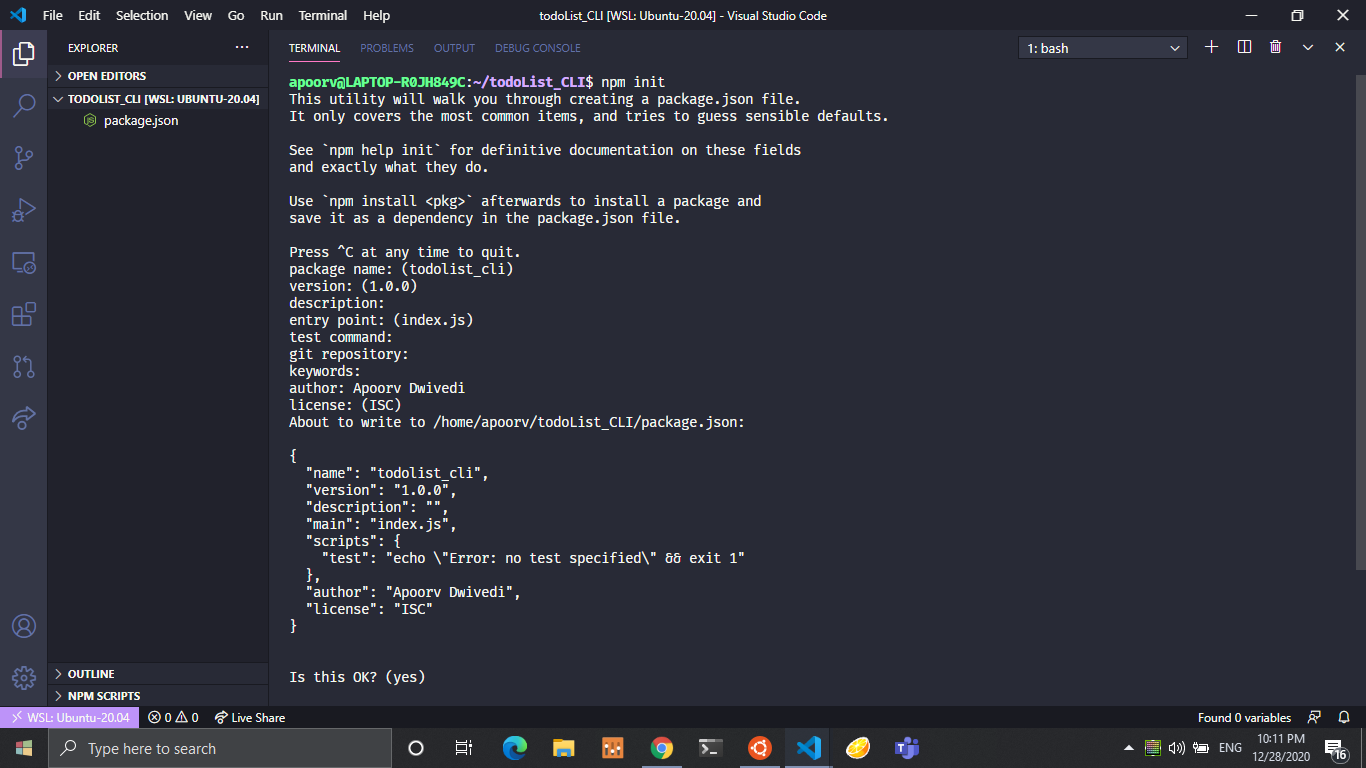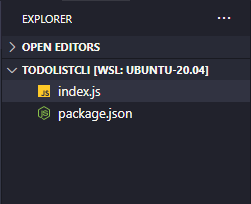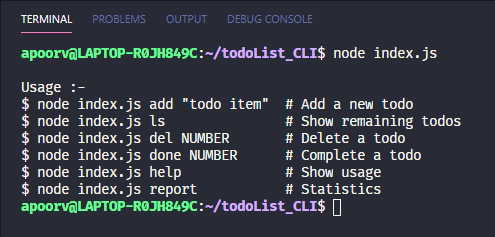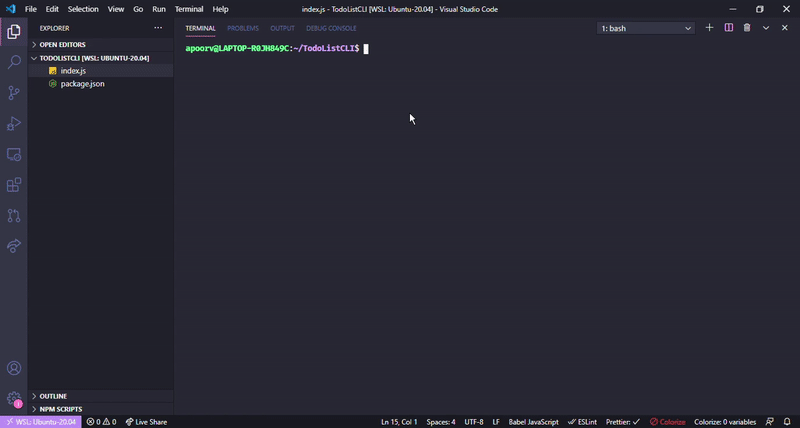GeeksforGeeks App
Open AppBrowser
Continue

# Todo List CLI application using Node.js

CLI is a very powerful tool for developers. We will be learning how to create a simple Todo List application for command line. We have seen TodoList as a beginner project in web development and android development but a CLI app is something we don’t often hear about.

Pre-requisites:

• A text editor, for example, VSCode, atom, etc.
• NPM should be installed.
• Basic understanding of JavaScript, es6 features, etc.

Create a new directory by mkdir <dir-name> and initiate an empty node project by cd into the directory and type the command npm init.Note: We won’t be using any third-party module in this project.

Project Directory: Now the project directory should consist of two files, package.json, and index.js as shown below:Some basic functionalities in our Todo List App will be the following:### Implementation:

Create an index.js file and write down the following code into it

## Javascript

 `// Requiring module``const fs = require(``'fs'``);`` ` `// Accessing arguments``const args = process.argv;`` ` `// The "index.js" is 8 characters long ``// so -8 removes last 8 characters``const currentWorkingDirectory = args.slice(0, -8);`

Explanation: We will be writing todo Tasks in a file called todo.txt and when they will be completed they will be erased from todo.txt and will be written in done.txt. The report will show how many todo has been completed and how many are left. To start with, we will import the fs module which will give us access to the file system.

Then, we will get the arguments passed using process.argv and store them in a variable named args. The process.argv returns an array in which there is the location of node modules, current working directory, and further arguments passed. We will retrieve the cwd by removing the name of the file.

Next, we will check if todo.txt and done.txt already exist in cwd, if not we will create them as shown below:

## Javascript

 `if` `(fs.existsSync(currentWorkingDirectory + ``'todo.txt'``) === ``false``) {``  ``let createStream = fs.createWriteStream(``'todo.txt'``);``  ``createStream.end();``}``if` `(fs.existsSync(currentWorkingDirectory + ``'done.txt'``) === ``false``) {``  ``let createStream = fs.createWriteStream(``'done.txt'``);``  ``createStream.end();``}`

Now we will create different functions for displaying usage, adding todo, deleting todo, etc. They will be called based on the arguments passed.

Info Function: It will display the usage format. It will be called when help is passed as an argument or when no argument is passed.

## Javascript

 `const InfoFunction = () => {``  ``const UsageText = ```Usage :-``\$ node index.js add ``"todo item"`  `# Add a new todo``\$ node index.js ls`               `# Show remaining todos``\$ node index.js del NUMBER`       `# Delete a todo``\$ node index.js done NUMBER`      `# Complete a todo``\$ node index.js help`             `# Show usage``\$ node index.js report`           `# Statistics`;`` ` `  ``console.log(UsageText);``};`

List Function: It will read the data from todo.txt and display them with a corresponding number. Most Recent is displayed on the top with the largest number.

## Javascript

 `const listFunction = () => {`` ` `  ``// Create a empty array``  ``let data = []; ``   ` `  ``// Read from todo.txt and convert it``  ``// into a string``  ``const fileData = fs.readFileSync(``    ``currentWorkingDirectory + ``'todo.txt'``)``  ``.toString(); ``   ` `  ``// Split the string and store into array``  ``data = fileData.split(``'\n'``); ``   ` `  ``// Filter the string for any empty lines in the file``  ``let filterData = data.filter(``function` `(value) {``    ``return` `value !== ``''``;``  ``}); ``   ` `  ``if` `(filterData.length === 0) {``    ``console.log(``'There are no pending todos!'``);``  ``}``   ` `  ``for` `(let i = 0; i < filterData.length; i++) {``    ``console.log((filterData.length - i) + ``'. '` `    ``+ filterData[i]);``  ``}``};`

Add Function: It will read the content from todo.txt, add the new todo, and then rewrite it in todo.txt.

Note: Since we don’t have the functionality to set the pointer position in the file while writing and reading files in JavaScript, so every time we add new data we will have to read the data, make the modifications, and then re-write it back.

## Javascript

 `const addFunction = () => {`` ` `  ``// New todo string argument is stored``  ``const newTask = args; ``   ` `  ``// If argument is passed``  ``if` `(newTask) { ``   ` `    ``// Create a empty array``    ``let data = []; `` ` `    ``// Read the data from file todo.txt and ``    ``// convert it in string``    ``const fileData = fs``      ``.readFileSync(currentWorkingDirectory + ``'todo.txt'``)``      ``.toString(); ``       ` `    ``// New task is added to previous data  ``    ``fs.writeFile(``      ``currentWorkingDirectory + ``'todo.txt'``,``      ``newTask + ``'\n'` `+ fileData, ``       ` `      ``function` `(err) {`` ` `        ``// Handle if there is any error``        ``if` `(err) ``throw` `err; ``         ` `        ``// Logs the new task added``        ``console.log(``'Added todo: "'` `+ newTask + ``'"'``); ``      ``},``    ``);``  ``} ``else` `{ `` ` `    ``// If argument was no passed``    ``console.log(``'Error: Missing todo string. Nothing added!'``);``  ``}``};`

Delete Function: It will read the data from todo.txt, remove the corresponding task, and re-write the data back in a file.

## Javascript

 `const deleteFunction = () => {`` ` `  ``// Store which index is passed``  ``const deleteIndex = args; ``   ` `  ``// If index is passed``  ``if` `(deleteIndex) { ``   ` `    ``// Create a empty array``    ``let data = []; ``     ` `    ``// Read the data from file and convert``    ``// it into string``    ``const fileData = fs``      ``.readFileSync(currentWorkingDirectory + ``'todo.txt'``)``      ``.toString(); ``       ` `    ``data = fileData.split(``'\n'``);``     ` `    ``// Filter the data for any empty lines``    ``let filterData = data.filter(``function` `(value) {``      ``return` `value !== ``''``; ``    ``});``     ` `    ``// If delete index is greater than no. of task ``    ``// or less than zero``    ``if` `(deleteIndex > filterData.length || deleteIndex <= 0) {``      ``console.log(``        ``'Error: todo #'` `+ deleteIndex ``            ``+ ``' does not exist. Nothing deleted.'``,``      ``);   ``    ``} ``else` `{`` ` `      ``// Remove the task``      ``filterData.splice(filterData.length - deleteIndex, 1); ``       ` `      ``// Join the array to form a string``      ``const newData = filterData.join(``'\n'``); ``       ` `      ``// Write the new data back in file``      ``fs.writeFile(``        ``currentWorkingDirectory + ``'todo.txt'``,``        ``newData, ``        ``function` `(err) {``          ``if` `(err) ``throw` `err;`` ` `          ``// Logs the deleted index``          ``console.log(``'Deleted todo #'` `+ deleteIndex); ``        ``},``      ``);``    ``}``  ``} ``else` `{ `` ` `    ``// Index argument was no passed``    ``console.log(``'Error: Missing NUMBER for deleting todo.'``);``  ``}``};`

Done Function: This function will read data from todo.txt, split it into an array, store the task to be marked as done, delete it from todo.txt, re-write the data back to todo.txt. Now we will write the deleted task we stored along with the current date in done.txt.

## Javascript

 `const doneFunction = () => {`` ` `  ``// Store the index passed as argument``  ``const doneIndex = args; ``   ` `  ``// If argument is passed``  ``if` `(doneIndex) { ``   ` `    ``// Empty array``    ``let data = []; ``     ` `    ``// Create a new date object``    ``let dateobj = ``new` `Date(); ``     ` `    ``// Convert it to string and slice only the``    ``// date part, removing the time part``    ``let dateString = dateobj.toISOString().substring(0, 10); ``     ` `    ``// Read the data from todo.txt``    ``const fileData = fs``      ``.readFileSync(currentWorkingDirectory + ``'todo.txt'``)``      ``.toString(); ``     ` `    ``// Read the data from done.txt``    ``const doneData = fs``      ``.readFileSync(currentWorkingDirectory + ``'done.txt'``)``      ``.toString(); ``       ` `    ``// Split the todo.txt data``    ``data = fileData.split(``'\n'``); ``     ` `    ``// Filter for any empty lines``    ``let filterData = data.filter(``function` `(value) {``      ``return` `value !== ``''``;``    ``}); ``     ` `    ``// If done index is greater than no. of task or <=0``    ``if` `(doneIndex > filterData.length || doneIndex <= 0) {``      ``console.log(``'Error: todo #'` `          ``+ doneIndex + ``' does not exist.'``);``    ``} ``else` `{`` ` `      ``// Delete the task from todo.txt``      ``// data and store it``      ``const deleted = filterData.splice(``          ``filterData.length - doneIndex, 1);``       ` `      ``// Join the array to create a string``      ``const newData = filterData.join(``'\n'``); ``       ` `      ``// Write back the data in todo.txt``      ``fs.writeFile(``        ``currentWorkingDirectory + ``'todo.txt'``,``        ``newData, ``        ``function` `(err) {``          ``if` `(err) ``throw` `err;``        ``},``      ``);``       ` `      ``// Write the stored task in done.txt``      ``// along with date string``      ``fs.writeFile( ``        ``currentWorkingDirectory + ``'done.txt'``,``        ``'x '` `+ dateString + ``' '` `+ deleted ``         ``+ ``'\n'` `+ doneData,``        ``function` `(err) {``          ``if` `(err) ``throw` `err;``          ``console.log(``'Marked todo #'` `            ``+ doneIndex + ``' as done.'``);``        ``},``      ``);``    ``}``  ``} ``else` `{ `` ` `    ``// If argument was not passed``    ``console.log(``'Error: Missing NUMBER for'``        ``+ ``' marking todo as done.'``);``  ``}``};`

Report Function: It will read data from todo.txt and done.txt, calculate the number of tasks in each and display how many tasks are completed and how many are pending.

## Javascript

 `const reportFunction = () => {`` ` `    ``// Create empty array for data of todo.txt``    ``let todoData = [];`` ` `    ``// Create empty array for data of done.txt``    ``let doneData = [];`` ` `    ``// Create a new date object``    ``let dateobj = ``new` `Date();`` ` `    ``// Slice the date part``    ``let dateString = dateobj.toISOString().substring(0, 10);`` ` `    ``// Read data from both the files``    ``const todo = fs.readFileSync(currentWorkingDirectory``                ``+ ``'todo.txt'``).toString();``    ``const done = fs.readFileSync(currentWorkingDirectory``                ``+ ``'done.txt'``).toString();`` ` `    ``// Split the data from both files``    ``todoData = todo.split(``'\n'``);`` ` `    ``doneData = done.split(``'\n'``);``    ``let filterTodoData = todoData.filter(``function``(value) {``        ``return` `value !== ``''``;``    ``});`` ` `    ``let filterDoneData = doneData.filter(``function``(value) {`` ` `        ``// Filter both the data for empty lines``        ``return` `value !== ``''``;``    ``});`` ` `    ``console.log(``        ``dateString +``        ``' '` `+``        ``'Pending : '` `+``        ``filterTodoData.length +``        ``' Completed : '` `+``        ``filterDoneData.length,``        ``// Log the stats calculated``    ``);``};`

Now Since we have created all the functions, Now we will just put a switch statement and call functions based on arguments passed.

## Javascript

 `switch` `(args) {``  ``case` `'add'``: {``    ``addFunction();``    ``break``;``  ``}`` ` `  ``case` `'ls'``: {``    ``listFunction();``    ``break``;``  ``}`` ` `  ``case` `'del'``: {``    ``deleteFunction();``    ``break``;``  ``}``   ` `  ``case` `'done'``: {``    ``doneFunction();``    ``break``;``  ``}``   ` `  ``case` `'help'``: {``    ``InfoFunction();``    ``break``;``  ``}``   ` `  ``case` `'report'``: {``    ``reportFunction();``    ``break``;``  ``}``   ` `  ``default``: {``    ``InfoFunction();``    ``// We will display help when no ``    ``// argument is passed or invalid``    ``// argument  is passed``  ``}``}`

Filename: index.js Our final index.js file will look like the following:

## Javascript

 `const fs = require(``'fs'``);`` ` `const args = process.argv;`` ` `// The "index.js" is 8 characters long so -8``// removes last 8 characters``const currentWorkingDirectory = args.slice(0, -8);`` ` ` ` `if` `(fs.existsSync(currentWorkingDirectory +``        ``'todo.txt'``) === ``false``) {``    ``let createStream = fs.createWriteStream(``'todo.txt'``);``    ``createStream.end();``}``if` `(fs.existsSync(currentWorkingDirectory +``        ``'done.txt'``) === ``false``) {``    ``let createStream = fs.createWriteStream(``'done.txt'``);``    ``createStream.end();``}`` ` `const InfoFunction = () => {``    ``const UsageText = ```Usage :-``\$ node index.js add ``"todo item"`  `# Add a new todo``\$ node index.js ls`               `# Show remaining todos``\$ node index.js del NUMBER`       `# Delete a todo``\$ node index.js done NUMBER`      `# Complete a todo``\$ node index.js help`             `# Show usage``\$ node index.js report`           `# Statistics`;`` ` `    ``console.log(UsageText);``};`` ` `const listFunction = () => {`` ` `    ``// Create a empty array``    ``let data = [];`` ` `    ``// Read from todo.txt and convert it into a string``    ``const fileData = fs``        ``.readFileSync(currentWorkingDirectory +``            ``'todo.txt'``).toString();`` ` `    ``// Split the string and store into array``    ``data = fileData.split(``'\n'``);`` ` `    ``// Filter the string for any empty lines in the file``    ``let filterData = data.filter(``function``(value) {``        ``return` `value !== ``''``;``    ``});`` ` `    ``if` `(filterData.length === 0) {``        ``console.log(``'There are no pending todos!'``);``    ``}``    ``for` `(let i = 0; i < filterData.length; i++) {``        ``console.log((filterData.length - i) + ``'. '` `+``            ``filterData[i]);``    ``}``};`` ` `const addFunction = () => {`` ` `    ``// New todo string argument is stored``    ``const newTask = args;`` ` `    ``// If argument is passed``    ``if` `(newTask) {`` ` `        ``// create a empty array``        ``let data = [];`` ` `        ``// Read the data from file todo.txt and``        ``// convert it in string``        ``const fileData = fs``            ``.readFileSync(currentWorkingDirectory +``                ``'todo.txt'``).toString();`` ` `        ``// New task is added to previous data``        ``fs.writeFile(``            ``currentWorkingDirectory + ``'todo.txt'``,``            ``newTask + ``'\n'` `+ fileData,`` ` `            ``function``(err) {`` ` `                ``// Handle if there is any error``                ``if` `(err) ``throw` `err;`` ` `                ``// Logs the new task added``                ``console.log(``'Added todo: "'` `+ newTask + ``'"'``);``            ``},``        ``);``    ``} ``else` `{`` ` `        ``// If argument was no passed``        ``console.log(``'Error: Missing todo string.'` `+``            ``' Nothing added!'``);``    ``}``};`` ` `const deleteFunction = () => {`` ` `    ``// Store which index is passed``    ``const deleteIndex = args;`` ` `    ``// If index is passed``    ``if` `(deleteIndex) {`` ` `        ``// Create a empty array``        ``let data = [];`` ` `        ``// Read the data from file and convert``        ``// it into string``        ``const fileData = fs``            ``.readFileSync(currentWorkingDirectory +``                ``'todo.txt'``).toString();`` ` `        ``data = fileData.split(``'\n'``);``        ``let filterData = data.filter(``function``(value) {`` ` `            ``// Filter the data for any empty lines``            ``return` `value !== ``''``;``        ``});`` ` `        ``// If delete index is greater than no. of task``        ``// or less than zero``        ``if` `(deleteIndex > filterData.length || deleteIndex <= 0) {``            ``console.log(``                ``'Error: todo #'` `+ deleteIndex +``                ``' does not exist. Nothing deleted.'``,``            ``);`` ` `        ``} ``else` `{``             ` `            ``// Remove the task``            ``filterData.splice(filterData.length - deleteIndex, 1);``             ` `            ``// Join the array to form a string``            ``const newData = filterData.join(``'\n'``);``             ` `            ``// Write the new data back in file``            ``fs.writeFile(``                ``currentWorkingDirectory + ``'todo.txt'``,``                ``newData, ``                ``function``(err) {``                    ``if` `(err) ``throw` `err;`` ` `                    ``// Logs the deleted index``                    ``console.log(``'Deleted todo #'` `+ deleteIndex);``                ``},``            ``);``        ``}``    ``} ``else` `{`` ` `        ``// Index argument was no passed``        ``console.log(``'Error: Missing NUMBER for deleting todo.'``);``    ``}``};`` ` `const doneFunction = () => {``     ` `    ``// Store the index passed as argument``    ``const doneIndex = args;``     ` `    ``// If argument is passed``    ``if` `(doneIndex) {``         ` `        ``// Empty array``        ``let data = [];``         ` `        ``// Create a new date object``        ``let dateobj = ``new` `Date();``         ` `        ``// Convert it to string and slice only the``        ``//  date part, removing the time part``        ``let dateString = dateobj.toISOString()``                    ``.substring(0, 10);``         ` `        ``// Read the data from todo.txt``        ``const fileData = fs``            ``.readFileSync(currentWorkingDirectory``                ``+ ``'todo.txt'``).toString();``         ` `        ``// Read the data from done.txt``        ``const doneData = fs``            ``.readFileSync(currentWorkingDirectory``                ``+ ``'done.txt'``).toString();``         ` `        ``// Split the todo.txt data``        ``data = fileData.split(``'\n'``);``         ` `        ``// Filter for any empty lines``        ``let filterData = data.filter(``function``(value) {``            ``return` `value !== ``''``;``        ``});``         ` `        ``// If done index is greater than``        ``// no. of task or <=0``        ``if` `(doneIndex > filterData.length || doneIndex <= 0) {``            ``console.log(``'Error: todo #'` `+ doneIndex ``                    ``+ ``' does not exist.'``);``             ` `        ``} ``else` `{``             ` `            ``// Delete the task from todo.txt data``            ``// and store it``            ``const deleted = filterData.splice(``                ``filterData.length - doneIndex, 1);``             ` `            ``// Join the array to create a string``            ``const newData = filterData.join(``'\n'``);``             ` `            ``// Write back the data in todo.txt``            ``fs.writeFile(``                ``currentWorkingDirectory + ``'todo.txt'``,``                ``newData,``                 ` `                ``function``(err) {``                    ``if` `(err) ``throw` `err;``                ``},``            ``);``            ``fs.writeFile(`` ` `                ``// Write the stored task in done.txt``                ``// along with date string``                ``currentWorkingDirectory + ``'done.txt'``,``                ``'x '` `+ dateString + ``' '` `+ deleted ``                                ``+ ``'\n'` `+ doneData,``                ``function``(err) {``                    ``if` `(err) ``throw` `err;``                    ``console.log(``'Marked todo #'` `                        ``+ doneIndex + ``' as done.'``);``                ``},``            ``);``        ``}``    ``} ``else` `{``        ``// If argument was not passed``        ``console.log(``'Error: Missing NUMBER for '``                ``+  ``'marking todo as done.'``);``    ``}``};`` ` `const reportFunction = () => {``     ` `    ``// Create empty array for data of todo.txt``    ``let todoData = [];``     ` `    ``// Create empty array for data of done.txt``    ``let doneData = [];``     ` `    ``// Create a new date object``    ``let dateobj = ``new` `Date();``     ` `    ``// Slice the date part``    ``let dateString = dateobj.toISOString()``                    ``.substring(0, 10);``     ` `    ``// Read data from both the files``    ``const todo = fs.readFileSync(``            ``currentWorkingDirectory ``            ``+ ``'todo.txt'``).toString();`` ` `    ``const done = fs.readFileSync(``        ``currentWorkingDirectory ``        ``+ ``'done.txt'``).toString();`` ` `    ``// Split the data from both files``    ``todoData = todo.split(``'\n'``);``     ` `    ``doneData = done.split(``'\n'``);``    ``let filterTodoData = todoData.filter(``function``(value) {``        ``return` `value !== ``''``;``    ``});``    ``let filterDoneData = doneData.filter(``function``(value) {``        ``return` `value !== ``''``;``        ``// Filter both the data for empty lines``    ``});``    ``console.log(``        ``dateString +``        ``' '` `+``        ``'Pending : '` `+``        ``filterTodoData.length +``        ``' Completed : '` `+``        ``filterDoneData.length,``        ``// Log the stats calculated``    ``);``};`` ` `switch` `(args) {``    ``case` `'add'``:``        ``{``            ``addFunction();``            ``break``;``        ``}`` ` `    ``case` `'ls'``:``        ``{``            ``listFunction();``            ``break``;``        ``}`` ` `    ``case` `'del'``:``        ``{``            ``deleteFunction();``            ``break``;``        ``}`` ` `    ``case` `'done'``:``        ``{``            ``doneFunction();``            ``break``;``        ``}`` ` `    ``case` `'help'``:``        ``{``            ``InfoFunction();``            ``break``;``        ``}`` ` `    ``case` `'report'``:``        ``{``            ``reportFunction();``            ``break``;``        ``}`` ` `    ``default``:``        ``{``            ``InfoFunction();``            ``// We will display help when no ``            ``// argument is passed or invalid``            ``// argument  is passed``        ``}``}`

Step to run the application:

Run the index.js file using the following command:

`node index.js`

Output:My Personal Notes arrow_drop_up
Related Tutorials